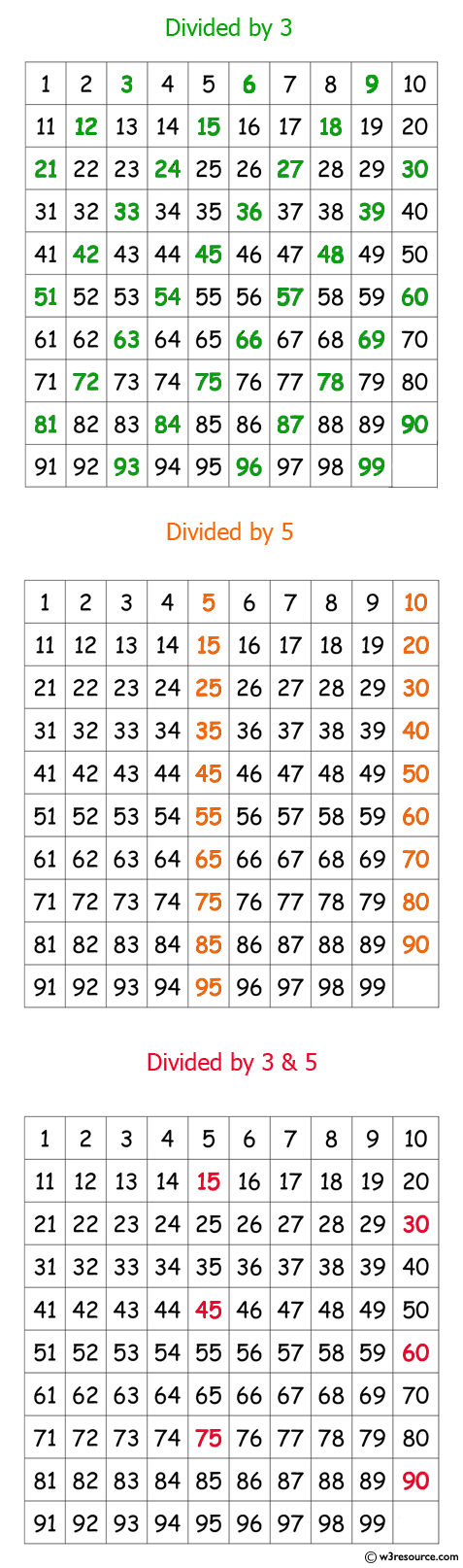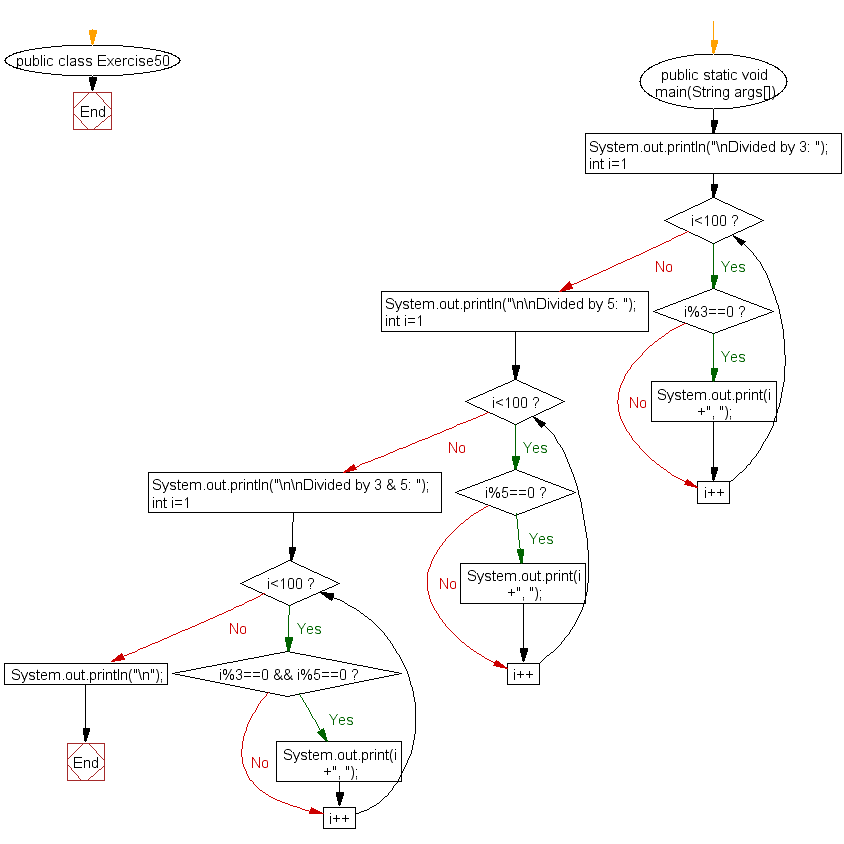﻿ Java: Numbers between 1 to 100, divisible by 3, 5 and both# Java Exercises: Print numbers between 1 to 100 which are divisible by 3, 5 and by both

## Java Basic: Exercise-50 with Solution

Write a Java program to print numbers between 1 to 100 which are divisible by 3, 5 and by both.

Pictorial Presentation:Sample Solution:

Java Code:

``````public class Exercise50 {
public static void main(String args[]) {
System.out.println("\nDivided by 3: ");
for (int i=1; i<100; i++) {
if (i%3==0)
System.out.print(i +", ");
}

System.out.println("\n\nDivided by 5: ");
for (int i=1; i<100; i++) {
if (i%5==0) System.out.print(i +", ");
}

System.out.println("\n\nDivided by 3 & 5: ");
for (int i=1; i<100; i++) {
if (i%3==0 && i%5==0) System.out.print(i +", ");
}
System.out.println("\n");
}
}
```
```

Sample Output:

```Divided by 3:
3, 6, 9, 12, 15, 18, 21, 24, 27, 30, 33, 36, 39, 42, 45, 48, 51, 54, 57
, 60, 63, 66, 69, 72, 75, 78, 81, 84, 87, 90, 93, 96, 99,

Divided by 5:
5, 10, 15, 20, 25, 30, 35, 40, 45, 50, 55, 60, 65, 70, 75, 80, 85, 90,
95,

Divided by 3 & 5:
15, 30, 45, 60, 75, 90,
```

Flowchart:Java Code Editor:

What is the difficulty level of this exercise?

Test your Programming skills with w3resource's quiz.

﻿

## Java: Tips of the Day

countOccurrences

Counts the occurrences of a value in an array.

Use Arrays.stream().filter().count() to count total number of values that equals the specified value.

```public static long countOccurrences(int[] numbers, int value) {
return Arrays.stream(numbers)
.filter(number -> number == value)
.count();
}
```

Ref: https://bit.ly/3kCAgLb